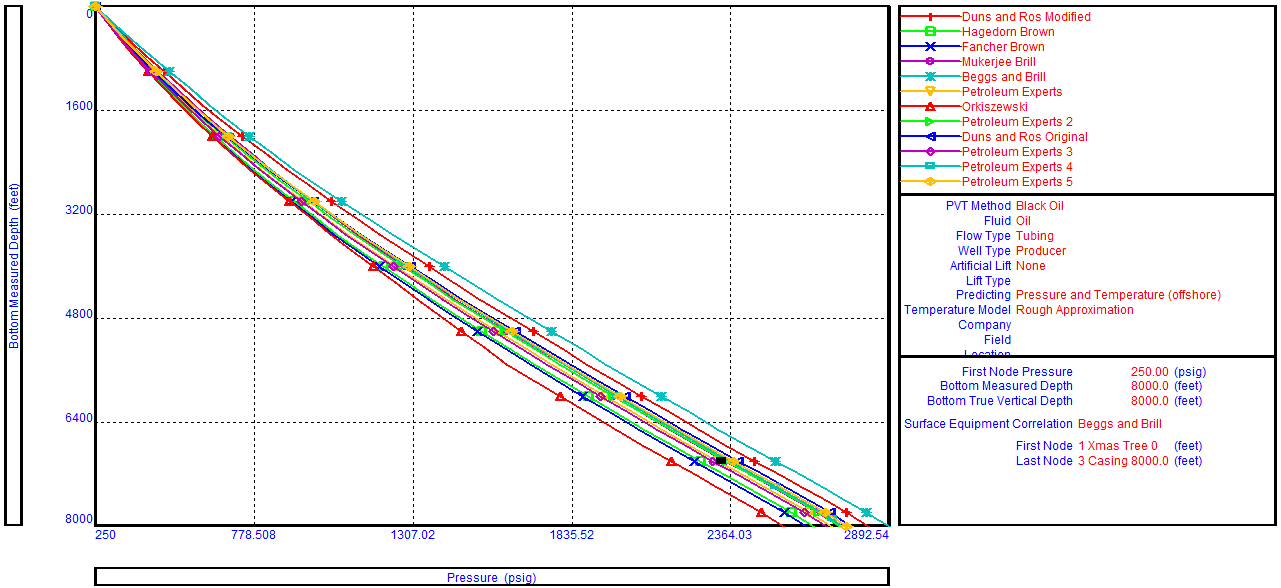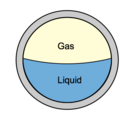## Multiphase flow correlations

The primary purpose of a multiphase flow correlations is to predict the liquid holdup (and hence the flowing mixture density) and the frictional pressure gradient.  This article details the most widely used correlations for the prediction of the Vertical Lift Performance.

The oil and water are lumped together as one equivalent fluid. Thus flow correlations in common use consider liquid/gas interactions.  They are therefore more correctly termed two-phase flow correlations.  Depending on the particular correlation, flow regimes are identified and specialized holdup and friction gradient calculations are applied for each flow regime.

There is no universal rule for selecting the best flow correlation for a given application. When an outflow performance simulator is used, it is recommended that a Correlation Comparison always be carried out. By inspecting the predicted flow regimes and pressure results, the User can select the correlation that best models the physical situation.## Multiphase flow correlations:

### Fancher & Brown:

• Fancher and Brown is a no-slip correlation, with no flow regime map. Therefore, this correlation cannot be recommended for general use and it is provided for use as a quality control (should not be used for quantitative work).
• It gives the lowest possible value of Vertical lift Performance (VLP). Therefore, Measured data falling to the left of Fancher Brown on the correlation comparison plot indicates a problem with fluid density (i.e PVT) or field pressure data.
• According to Brown, it is only suitable for 2-3/8 – 2-7/8 inch tubing.
• It is for GLR less than 5000 scf/bbl and flow rates less than 400 bpd.
• It has its own friction factor model, which is independent of pipe roughness.

## Multiphase Flow Properties & Pressure Gradient Calculation

For oil wells, the main component of pressure loss is the gravity or hydrostatic term.  Calculation of the hydrostatic pressure loss requires knowledge of the proportion of the pipe occupied by liquid (holdup) and the densities of the liquid and gas phases.  Accurate modeling of fluid PVT properties is essential to obtain in-situ gas/liquid proportions, phase densities, and viscosities.Calculation of holdup is complicated by the phenomenon of gas/liquid slip.  Gas, being less dense than liquid flows with a greater vertical velocity than liquid. The difference in velocity between the gas and liquid is termed the slip velocity.  The effect of slip is to increase the mixture density and hence the gravity pressure gradient.

In the next paragraphs, two-phase flow properties (holdup, densities, velocity, and viscosity) will be detailed. Then the pressure gradient equation which is applicable to any fluid flowing in a pipe inclined at an angle φ is depicted. As well as, the two-phase flow procedure to calculate the outlet pressure is detailed.

## Two-Phase Flow Properties:

### Holdup:

With reference to multiphase flow in pipes, the fraction of a particular fluid present in an interval of a pipe. In multiphase flow, each fluid moves at a different speed due to different gravitational forces and other factors, with the heavier phase moving slower, or being more held up, than the lighter phase. The holdup of a particular fluid is not the same as the proportion of the total flow rate due to that fluid, also known as its ”cut”. To determine in-situ flow rates, it is necessary to measure the holdup and velocity of each fluid. The sum of the holdups of the fluids present is unity.

### 1- Liquid and Gas Holdup (HL & Hg):

HL is defined as the ratio of the volume of a pipe segment occupied by the liquid to the volume of the pipe segment. The remainder of the pipe segment is of course occupied by gas, which is referred to as Hg.

Hg = 1 – HL